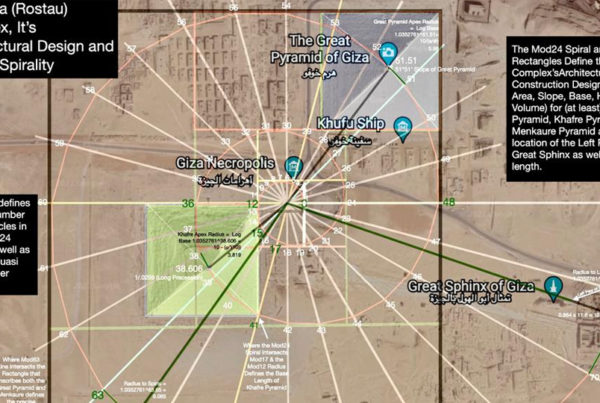"Do not go where the path may lead, go instead where there is no path and leave a trail"

— Ralph Waldo Emerson

"Do not go where the path may lead, go instead where there is no path and leave a trail"

— Ralph Waldo Emerson

### A Novel Geometric Model of Natural Spirals Based on Right Triangle Polygonal Modular Formations

In this paper we propose a novel class of spirals that are based on perfect polygonal formations. The spirals are defined by a fractal of right triangles that delineate their geometry and determine their progression rates and modes. We show how these spirals match naturally occurring ones, including hurricanes and galaxies, much better than already proposed models, allowing for more accurate categorization of these phenomena and better prediction of their physical properties.

### Icositetragon Wheel

Recently, a 24-based distribution of numbers was used in a novel method for an infinite and accurate prime prediction and factorization. Therefore, further investigation of this particular configuration of numbers, labeled here the icositetragon wheel, is essential if we to expand our understanding of this method and to further improve it. We will show that using the icositetragon wheel is not an arbitrary choice by elaborating on the unique properties this configuration has, not only in regard to prime numbers distribution, but also for the many symmetries and complementary properties that numbers, prime and non-prime, observe in it.

### Precise Geometrical Correspondence to the Perfect 5th Tuned to the 432Hz Frequency

In this paper, we show how the 12 notes of the octave have inherent decimal references that correspond precisely to the internal angles of regular polygonal shapes that exhibit symmetries found abundantly in nature. This exact correspondence manifests itself only when the standard pitch tuning is set to 432Hz instead of the modern 440Hz, which is an indicator on the importance of this tuning, from a mathematical perspective at least.

### Prime Number Pattern and Discovery of Quasi-Prime

When integers are continuously plotted around each side of an icositetragon (24-side polygon), patterns of primes and a new classification of prime numbers (Quasi-primes) emerge.

### Predicting Prime Numbers

When integers are continuously plotted around each side of an icositetragon (24-sided polygon), patterns of primes and a new classification of prime numbers (Quasi-primes) emerge. This information presents a method to predict prime number incidence.

### Quaternion Symmetry of the Icositetragon

When integers are continuously plotted around each side of an icositetragon (24-sided polygon), quaternion sets are demonstrated, allowing the identification of charge-associated quadripolarity.

### Unified Mathematics, Geometry and Music

Unified mathematical models must establish numerical connections between all types of physical phenomenon. Inherent relationships between geometry and music are shown through the inscription of regular polygons within a unit circle.

### Accurate and Infinite Prime Prediction from Novel Quasi-Prime Analytical Methodology

It is known that prime numbers occupy specific geometrical patterns or moduli when numbers from one to infinity are distributed around polygons having sides that are integer multiple of number 6. In this paper, we will show that not only prime numbers occupy these moduli, but non-prime numbers sharing these same moduli have unique prime-ness properties. When utilizing digital root methodologies, these non-prime numbers provide a novel method to accurately identify prime numbers and prime factors without trial division or probabilistic-based methods.

### Four-Fold Mirror-Symmetry Inherent to the Icositetragon Distribution of Numbers

Recently, a 24-based distribution of numbers was used in a novel method for an infinite and accurate prime prediction and factorization. Therefore, further investigation of this particular configuration of numbers, labeled here the icositetragon wheel, is essential if we are to expand our understanding of this method and to further improve it. We will show that using the icositetragon wheel is not an arbitrary choice by elaborating on the unique properties this configuration has, not only in regard to prime numbers distribution, but also for the many symmetries and complementary properties that numbers, prime and non-prime, observe in it.

### The Wave Theory of Numbers

The concept of waves is very fundamental to classical and modern physics alike, being essential in describing light, sound, and elementary particles, among many other phenomena. In this paper, we show that the wave/particle duality is a phenomenon manifested not only in the physical world and the mathematics that describes it but also in the simple numbers that form the basic matrix upon which most of our sciences rest. We will also show how this wave-based approach to numbers could be essential to our understanding of the mathematical and physical constants that govern the physical laws as well as the natural elements emerging from them.

### The Wave Theory of Constants

Guided by the wave theory of numbers, we examine the fundamental constants of nature through the unique characteristics of its wave-matrix. The fractal nature of the matrix, with its 12-based periodicity, forms the kernels from which constants are generated thus enabling a better understanding of their origin as well as their unique numerical sequencing. Additionally, the inherent numerical and geometrical symmetries of the matrix will prove to be a powerful tool for discovering new fundamental constants not yet known to the scientific community.

### The Fractal Root of Numbers

Formally, the principal roots of numbers, i.e. square or cube roots, etc., are believed to exclusively consist of numerically identical numbers. In this paper, we expand this formal definition by exploring the existence of fractal roots; roots that are identical in their numerical structure but not in their relative magnitude. This new type of root has its own mathematical and geometrical peculiarities with implications intersecting many fields of science.